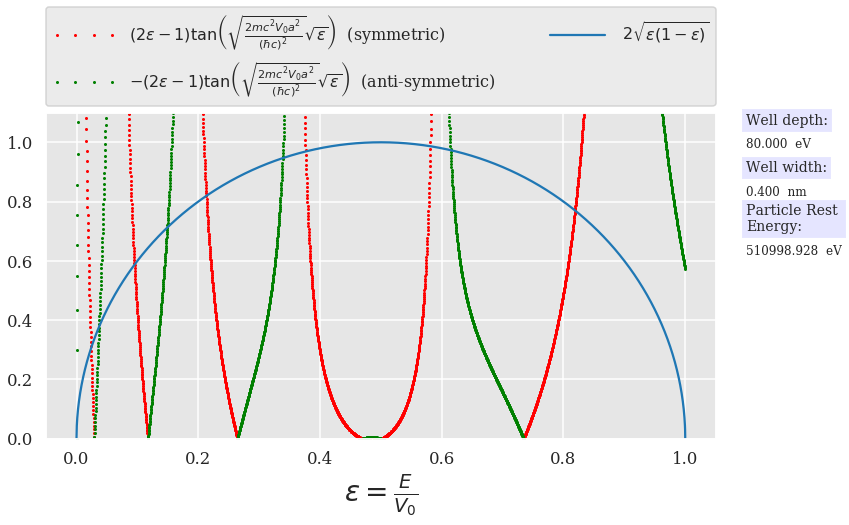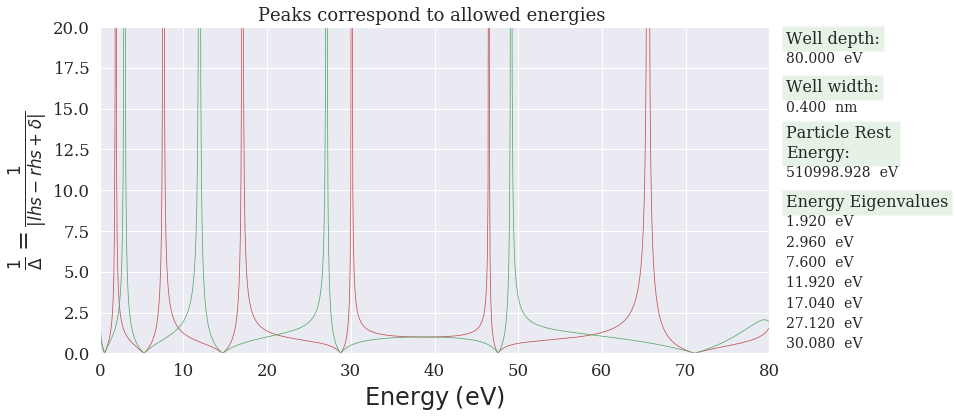# The Quantum Mechanical Finite Square Well: Bound State solutions¶

## Width = $a$, depth = $V_0$¶

Paul Nakroshis
Dept. of Physics, University of Southern Maine
pauln at maine dot edu
12 Feb 2019

In :
import numpy as np
import matplotlib.pyplot as plt
from scipy import signal
from pylab import rcParams
rcParams['figure.figsize'] = 10,8
from scipy.signal import argrelextrema as findpeaks
import seaborn as sns   # makes pretty plots
%matplotlib inline
#sns.set_style("darkgrid", {"grid.linewidth": .5, "axes.facecolor": ".9"})
#sns.set_context("notebook", font_scale=1.5, rc={"lines.linewidth": 2.5})
#sns.set_context("paper")
#sns.set_context("talk")


In this notebook, I look at the bound state solutions to the energy eigenvalues for a finite square well with width $a$ and depth $V_0$ as shown in the figure below:To find the solutions, we write down Schrodinger's equation $$- \frac{\hbar^2}{2m}\frac{d^2\psi}{dx^2} + V(x)\phi = E\psi$$ for the regions $x<0$, $0<x<a$, and $x>a$, and impose continuity of $\phi$ and its derivative at $x=0$ and $x=a$. When one works through this process (see any introductory quantum text) ones finds that allowed bound state soltutions satisfy the transcendental equation which I write as
$$\pm(2\epsilon -1)\tan\left(\sqrt{\frac{2 m c^2 a^2 V_0}{(\hbar c)^2}} \sqrt{\epsilon}\right) = 2\sqrt{\epsilon(1-\epsilon)}\;\;\;\;\;\;\;\;(eq.1)$$

where $\epsilon = E/V_0$ is the dimensionless energy which we want to find, and the positive sign corresponds to symmetric solutions and the negative to antisymmetric solutions (with respect to the center of the well). The standard way to find the allowed energy levels is to find the solutions to eq. 1 above by plotting the left side and the right side of the equations, and look for intersections; to do so, I'll define the left (symmetric and antisymmetric cases determined by a boolean; True = symmetric) and right sides here, and define the well as containing (by default) an electron and with a default width as 2 Bohr radii:

To solve this for a specific case, we define a function for each side of the equation

In :
def leftSide(ϵ, S = True, V = 50, a=2*0.052917721092, mc2=0.510998928e6):
"""
DESCRIPTION:
Calculates the left hand side of equation 1 above;
note that the argument of the tangent function contains
all the relevant features of the well. This function defaults
to
symmetry : S = True for symmetric solutions; S = False for antisymmetric
depth    : V = 50 eV
width    : a = 2*0.0529177 nm = 2 Bohr radii
particle rest energy: mc2 = 0.51099e+6 eV = electron rest energy

USAGE: This means, of course, that the user may call this function by

leftSide(ϵ)   # will use default well params

or

leftSide(ϵ, False, 20,0.20.5109989)  # will find Anti-symmetric soltions for
# V=20 eV, and a = 0.2 nm, and default mass energy

"""
#define needed constants for the well:
c = 299792458.0      # in m/s
hbar_c = 197.3269718 # in eV-nm

#now calculate the left hand side:
value = np.sqrt(2*mc2*V*a**2)/hbar_c
return (-1)**(S+1)*(2.*ϵ - 1.0)*np.tan(value*np.sqrt(ϵ))

def rightSide(ϵ):
#
#calculates the right hand side of equation 1 above
#
return 2*np.sqrt(ϵ *(1.0-ϵ))


### Standard Solution method: find intersection of curves¶

First, note that equation 1 is likely different than seen in standard texts in that I have placed the term $(2\epsilon -1)$ on the left hand side---whereas in many texts you'll see that term in the denominator of the right hand side. I've done so, because this term makes the right hand side pathalogical when $\epsilon\rightarrow\frac{1}{2}$, so by placing it on the left hand side, this issue is avoided.

The standard method for solving this is to plot both sides of equation 1 and look for the intersection points. In the next cell, I enter the parameters that describe the well, as well as an array of $N$ $\epsilon$ values evenly spaced from 0 to N.

In :
N = 4000
V = 80.0             # set well depth in eV
#a = 2*0.0529177     # set well width in nm
a = 0.4
mc2 = 0.510998928e6  # set particle rest energy in eV

ϵ = np.linspace(0,1.0,N) # create array of ϵ values to search

In :
## these lines set up plot style and limits
## I use the seaborn package 'cause it looks nice
sns.set_context("talk")
plt.figure(figsize=(12, 6))
sns.set_style("darkgrid", {"grid.linewidth": .6, "axes.facecolor": ".9",\
"font.family": 'serif',"font.serif": "Times"})
##
## here's the actual plotting command for each side of eq. 1:
##
## First symmetric soltions:
plt.plot(ϵ, leftSide(ϵ, True, V, a, mc2),'r.', markersize=4,\
label=r'$(2\epsilon - 1)\tan\left(\sqrt{\frac{2mc^2V_0 a^2}{(\hbar c)^2}}\sqrt{\epsilon}\right)\;$ (symmetric)')
## Then anti-symmetric solutions:
plt.plot(ϵ, leftSide(ϵ, False, V, a, mc2),'g.', markersize=4,\
label=r'$-(2\epsilon - 1)\tan\left(\sqrt{\frac{2mc^2V_0 a^2}{(\hbar c)^2}}\sqrt{\epsilon}\right)\;$ (anti-symmetric)')

plt.plot(ϵ ,rightSide(ϵ), label=r'$2\sqrt{\epsilon(1-\epsilon)}$')
##
## these lines set up plot limits
plt.ylim(0,1.1)
plt.xlabel(r'$\epsilon = \frac{E}{V_0}$', fontsize=28)
plt.legend(bbox_to_anchor=(0., 1.02, 1., .102), loc=3,
handlelength=4, frameon=True,fontsize=16, numpoints=4)
##
##  finally, put the well & particle properties on the plot so it is
##  well documented.
plt.text(1.1,1.06, "Well depth:" ,fontsize=14, bbox=dict(facecolor='blue', alpha=0.10))
plt.text(1.1,0.98, "%4.3f  eV" %  V ,fontsize=12)
plt.text(1.1,0.90, "Well width:" ,fontsize=14, bbox=dict(facecolor='blue', alpha=0.10))
plt.text(1.1,0.82, "%4.3f  nm" %  a ,fontsize=12)
plt.text(1.1,0.70, "Particle Rest \nEnergy:" ,fontsize=14, bbox=dict(facecolor='blue', alpha=0.10))
plt.text(1.1,0.62, "%4.3f  eV" %  mc2 ,fontsize=12)

plt.show()The plot above nicely shows the solutions; the values of $\epsilon$ that correspond to the intersection points gives the energy solutions. The problem with this is that we have to graphically find these points, or resort to numerical root finding. Instead, I'll find the solutions by plotting the reciprical of the difference between the two sides; i.e. I will plot the function 'peaks' defined as: $$\mathrm{peaks} = \left|\pm \, (2\epsilon -1)\tan\left(\sqrt{\frac{2 m c^2 a^2 V_0}{(\hbar c)^2}} \sqrt{\epsilon}\right) - 2\sqrt{\epsilon(1-\epsilon)} + \delta\, \right|^{\,-1}\;\;\;(eq.2)$$ where $\delta$ is a small constant to prevent the function from becoming pathological when the left and right sides of eq. 1 are equal.

After this, I'll use the scipy scipy.signal routine argrelextrema (which I imported at the top of this notebook as findpeaks). This routine finds the index value that corresponds to the maxima of eq. 2.

In :
#N = 5000
V = 80          # set well depth in eV
#a = 2*0.0529177    # set well width in nm
a = 0.4
mc2 = 0.510998928e6  # set particle rest energy in eV
ymax = 20          # this is an arbitrary cutoff for the vertical axis of the plot.
# if you change it, it will mess up the formatting of the
# text strings added to the plot. Don't mess with this unless you
# want to deal with this consequence.
δ = 1.0e-4    # this is the factor to keep the "peaks" functions from blowing up.
δϵ = 1.0e-3
#ϵ = np.linspace(0,1.0,N) # create array of ϵ values to search
ϵ = np.arange(0,1.0,δϵ)
N = len(ϵ)
print(N)
#sns.set_context("paper")
sns.set_context("notebook")
plt.figure(figsize=(12, 6))
sns.set(context='notebook', style='darkgrid', palette='deep', font='serif',\
font_scale=1.5, rc={"lines.linewidth": 2.5})
###
#
#
peaks_S = 1/abs(leftSide(ϵ,True,V,a,mc2)-rightSide(ϵ )+ δ)   # this takes 1/Δ(lhs-rhs + δ)
peaks_A = 1/abs(leftSide(ϵ,False,V,a,mc2)-rightSide(ϵ)+ δ)  # this takes 1/Δ(lhs-rhs + δ)

plt.plot(V*ϵ , peaks_S,'r', linewidth=0.75 )
plt.plot(V*ϵ , peaks_A,'g', linewidth=0.75 )

plt.ylim(0,ymax)
plt.xlim(0,V)
plt.xlabel(r'$\mathrm{Energy\;(eV)}$', fontsize=24)
plt.ylabel(r'$\frac{1}{\Delta} = \frac{1}{\left| lhs-rhs+\delta\right|}$', fontsize=24)

plt.title('Peaks correspond to allowed energies ')
# for local maxima (uses scipy: from scipy.signal import argrelextrema as findpeaks)
#
indices_S = findpeaks(peaks_S, np.greater)
indices_A = findpeaks(peaks_A, np.greater)
#
#
#   find peaks with scipy peak finder
#
peak_ind = signal.find_peaks_cwt(peaks_S, np.arange(1,8),min_snr=2)
peak_ind = peak_ind[1:-1]
print("Symmetrical Solutions: ", V*ϵ[peak_ind])

peak_ind = signal.find_peaks_cwt(peaks_A, np.arange(1,10),min_snr=2)
peak_ind = peak_ind[1:-1]
print("Asymmetrical Solutions: ", V*ϵ[peak_ind])
#
energies=np.empty(1) # create an empty numpy array for the energy solutions.
### the scipy peak finder returns an array of peak index values, but also a second element
### (This is why I interate over indices here:)

for i in indices_S:
energies = np.append(energies,V*ϵ[i])
print('Symmetric Energy(ϵ=%4.3f) = %4.3f eV' % (float(i/N), V*float(ϵ[i])))
for i in indices_A:
energies = np.append(energies,V*ϵ[i])
print('AntiSymmetric Energy(ϵ=%4.3f) = %4.3f eV' % (float(i/N), V*float(ϵ[i])))

np.set_printoptions(formatter={'float': '{: 0.3f}'.format})
energies = energies[1:]
energiesSorted = np.sort(energies, axis=None)

plt.text(V+2,0.95*ymax, "Well depth:" ,fontsize=16, bbox=dict(facecolor='green', alpha=0.10))
plt.text(V+2,0.89*ymax, "%4.3f  eV" %  V ,fontsize=14)
plt.text(V+2,0.80*ymax, "Well width:" ,fontsize=16, bbox=dict(facecolor='green', alpha=0.10))
plt.text(V+2,0.74*ymax, "%4.3f  nm" %  a ,fontsize=14)
plt.text(V+2,0.60*ymax, "Particle Rest \nEnergy:" ,fontsize=16, bbox=dict(facecolor='green', alpha=0.10))
plt.text(V+2,0.54*ymax, "%4.3f  eV" %  mc2 ,fontsize=14)
### Next part prints out (at most) the first 7 energy eigenvalues to the plot.
plt.text(V+2,0.45*ymax, "Energy Eigenvalues" ,fontsize=16, bbox=dict(facecolor='green', alpha=0.10))
for i in range(len(energiesSorted)):
if i<7:
plt.text(V+2,(0.39 - 0.0625*i)*ymax, "%4.3f  eV" %  energiesSorted[i] ,fontsize=14)

plt.savefig('EnergyLevels80eVWell.png',dpi = 150)

1000
Symmetrical Solutions:  [ 1.92  7.68 17.12 30.16 46.56 65.52 70.08]
Asymmetrical Solutions:  [ 3.04 11.92 27.12 49.2 ]
Symmetric Energy(ϵ=0.024) = 1.920 eV
Symmetric Energy(ϵ=0.095) = 7.600 eV
Symmetric Energy(ϵ=0.213) = 17.040 eV
Symmetric Energy(ϵ=0.376) = 30.080 eV
Symmetric Energy(ϵ=0.581) = 46.480 eV
Symmetric Energy(ϵ=0.819) = 65.520 eV
AntiSymmetric Energy(ϵ=0.037) = 2.960 eV
AntiSymmetric Energy(ϵ=0.149) = 11.920 eV
AntiSymmetric Energy(ϵ=0.339) = 27.120 eV
AntiSymmetric Energy(ϵ=0.484) = 38.720 eV
AntiSymmetric Energy(ϵ=0.615) = 49.200 eV
AntiSymmetric Energy(ϵ=0.993) = 79.440 eVThere you go! A relatively straightforward code to compute the allowed symmetric and antisymmetric bound state energies for a finite potential well.

Please let me know if you find any errors in this code and/or find it useful. pauln at maine dot edu